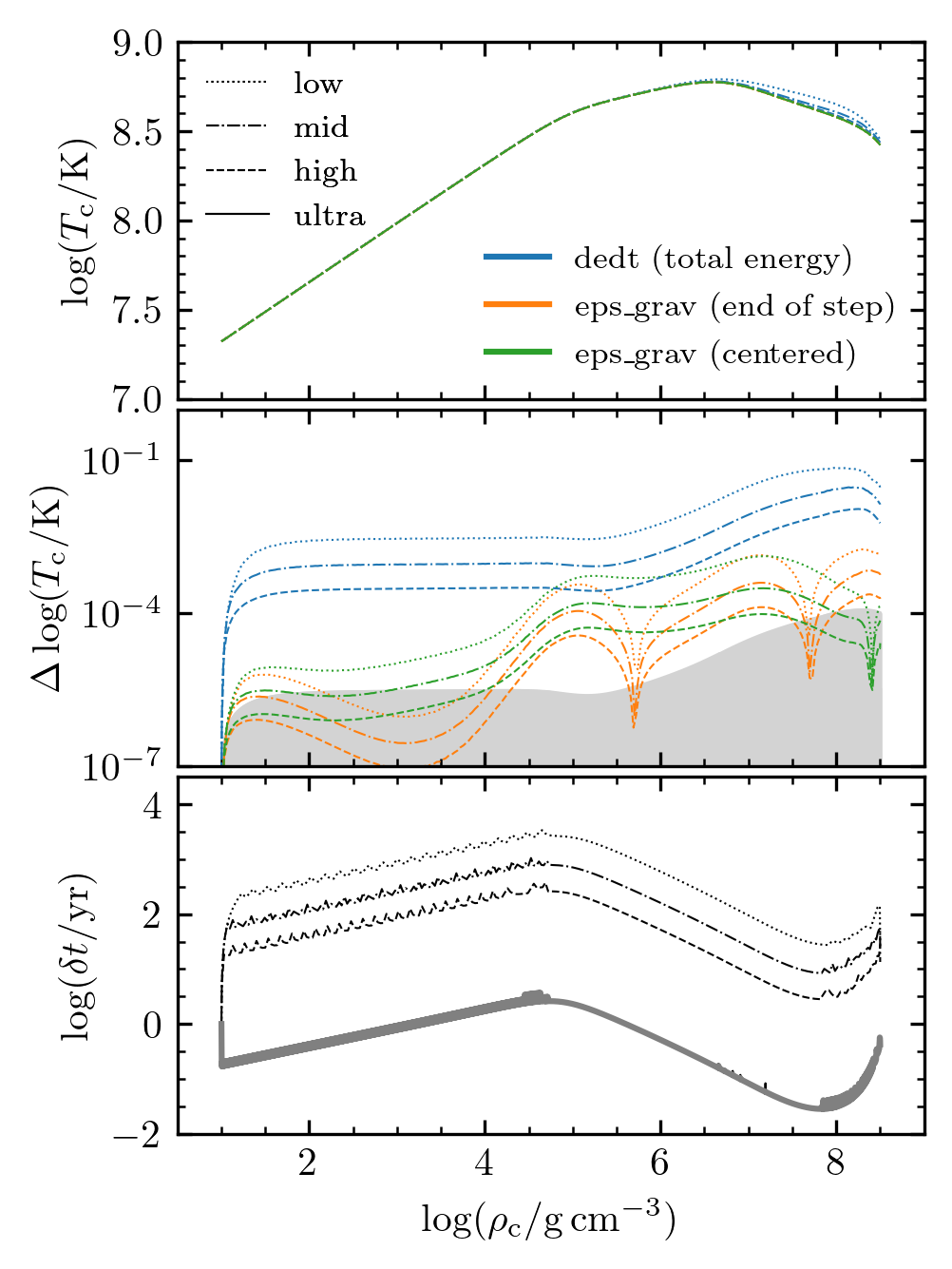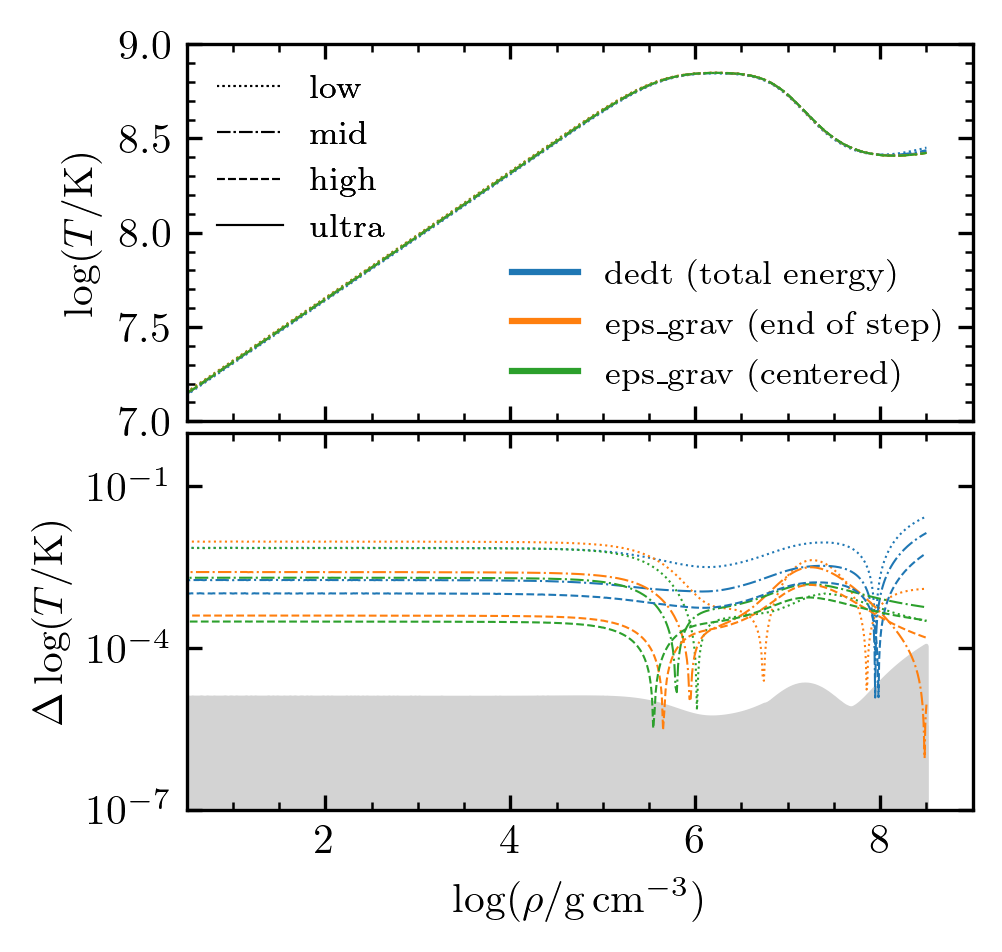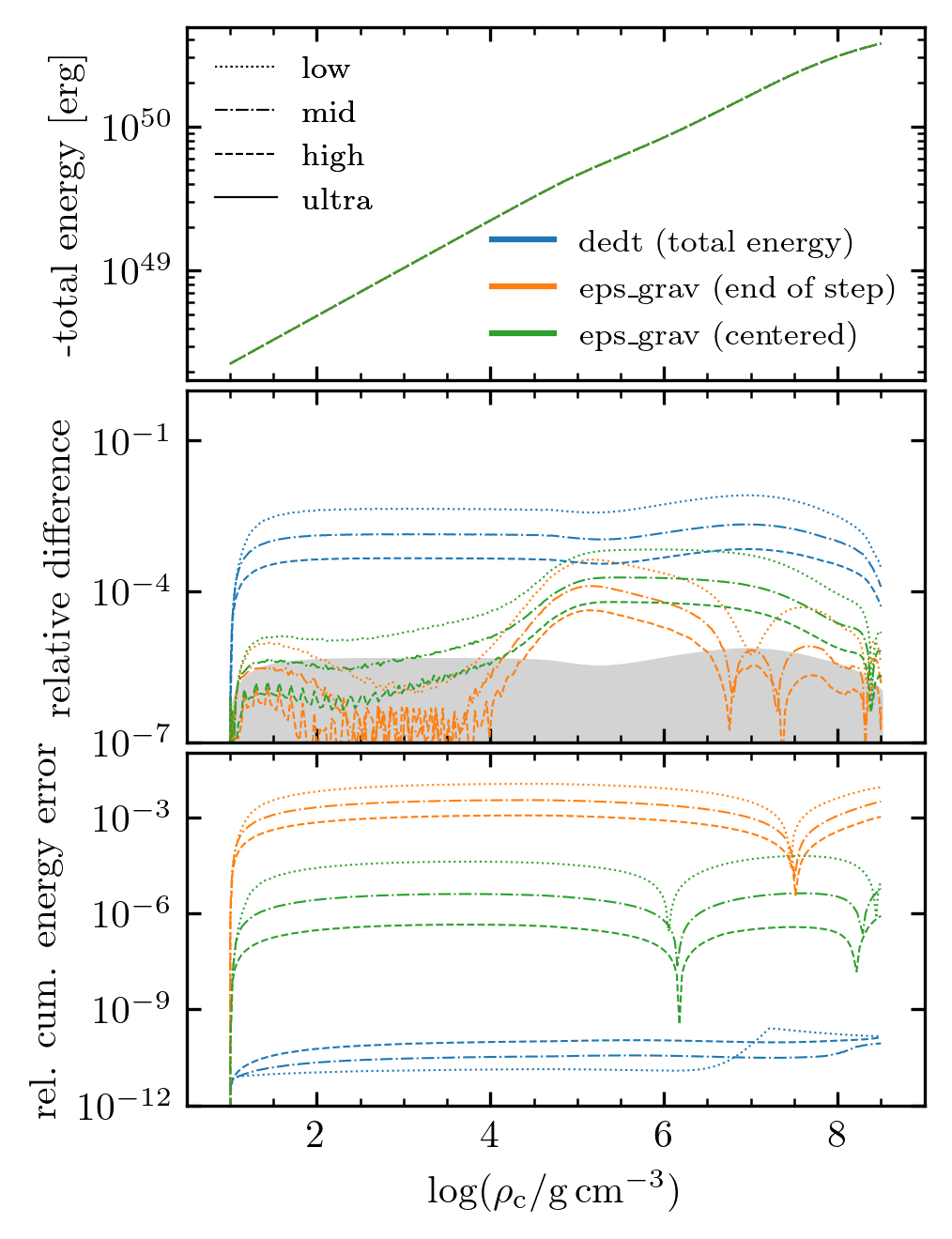# carbon_kh¶

This test case evolves an initially low-density, 1.3 $${\rm M}_\odot$$ stellar model with a pure carbon composition as it Kelvin-Helmholtz contracts.

The model begins at a central density of 10 $$\rm g\,cm^{-3}$$ and we follow the contraction over a more than 2 dex in decrease in radius and a more than 7 dex increase in central density. Nuclear reactions are not considered. For simplicity, we assume that the radiative opacities are given by electron scattering and include standard thermal neutrino losses. We exclusively use the HELM EOS, as the use of a single EOS that is formulated from the Helmholtz free energy avoids most of the EOS inconsistencies.

This case is not meant to model an object realized in nature, but provides a simple example problem that has neither mass changes nor composition changes. It is nonetheless demanding as the conditions in the star vary tremendously during the evolution as material goes from non-degenerate conditions to conditions of relativistic electron degeneracy and the dominant energy loss mechanism transitions from radiative diffusion to optically-thin neutrino cooling.

This case is discussed extensively in section 5.2 of MESA Instrument Paper VI. You can run the full resolution study described in that section by using the rnall bash script, which links to different energy equation and resolution options to run through the model 12 times. This will produce the output necessary for reproducing plots like the ones below and seen in figures 9 and 10 of the instrument paper.

By default, the test suite rn script runs just one of these instances: the high resolution run using the eps_grav energy equation option, which should run in a few minutes, appears reasonably converged based on the plots below, and exhibits a small cumulative energy error.

The included Jupyter notebook (docs/carbon_kh.ipynb) provides information about reproducing the following convergence study.Last-Updated: 2023-04-03 (mesa 81ad921) by Evan Bauer